# Describe closure concept in JavaScript

• Difficulty Level : Easy
• Last Updated : 24 Nov, 2021

In this article, you will learn about the concept of closure in Javascript & how to apply it on various functions. The closure in javascript is a way to combine all the functions & binds them in bundles with the lexical environment. We will deep dive into the closure concept as we grow in this article.

Prerequisite: Before we proceed to our main discussion, you should have a good working knowledge of javascript functions i.e. how memory allocation takes place, variable scope concept, the lexical environment in javascript.

Hey geek! The constant emerging technologies in the world of web development always keeps the excitement for this subject through the roof. But before you tackle the big projects, we suggest you start by learning the basics. Kickstart your web development journey by learning JS concepts with our JavaScript Course. Now at it's lowest price ever!

Let’s first start discussing the basic javascript program examples:

In case, if you don’t know how to add & execute the javascript in the HTML document, please refer to Where to put JavaScript in an HTML Document? article for further detailed knowledge.

We will quickly view that how the javascript code is being executed. For this, add the <script> tag in the index.html file for a reference to external javascript code or define the code inside the <script> tag. Subsequently, open the developer’s console tab on the browser to see the output of the code.

## index.html

 ```<``html``>`` ` `<``head``>``    ``<``title``>Closures```` ` `<``body``>``    ``<``h2``>Closures in Javascript``    ``<``script` `src``=``"./script.js"``>```` ` ``

Now, we will understand all the basic concepts like how the function works, how a function can be declared inside another function using the scope chain, returning the function from the function, through the examples, then will understand the closure concept.

Example 1: Here, we are simply illustrating the working of function as shown below:

## script.js

 `function` `a() {``  ``var` `x = 5;``  ``console.log(x);``}`` ` `function` `b() {``  ``var` `y = 15;``  ``console.log(y);``}``a();``b();`

Explanation:

1. First of all, a global execution context will be created and then the function definitions will get space in memory.
2. As soon as javascript encounters, a first function calls a(), it creates another execution context and reserves memory for variable x and puts undefined inside x.
3. Then the thread of execution phase comes into the picture and variable x gets the value 5 and in the next line console.log prints the value of x,  and finally when function execution finishes, it gets removed from the call stack and execution context in which variable x was stored gets destroyed, the same happens for function b().
4. But here we can’t access the variables x and y outside the block, because when the function finishes its execution context, an execution context also gets deleted and when the x is gone, it is nowhere in the memory. Same for function b(), we can’t access the var y outside that function.

Output: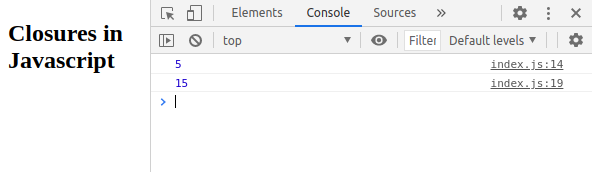Example 2: In this section, we will discuss the function inside the function using a scope chain to access the variable.

## script.js

 `function` `a() {``  ``var` `x = 5,``    ``y = 6;``  ``console.log(x);`` ` `  ``function` `b() {``    ``var` `z = 7;``    ``console.log(y);``    ``console.log(z);``  ``}``  ``b();``}``a();`

Explanation:

1. Here an execution context gets created and then function a() gets space inside the memory.
2. After then we call the function a(), so it gets its own execution context.
3. There is again a variable x and function b() which gets a space in memory and later during the code execution phase, variable x gets printed
4. As soon as control goes to call of function b(), this gets another execution context inside the last execution context and the var y gets space in memory after then it encounters a console.log(x) but x is not in the execution context of function b() so it goes to the parent lexical environment because of scope chain and hopefully finds it there and prints the value of x,
5. The var y is something that is inside the function hence gets printed without any extra effort.

Output: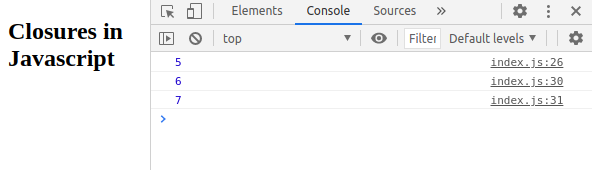Example 3: In this case, we will discuss the returning function from the function.

## script.js

 `function` `a() {``  ``console.log(``"Hello, I am a function"``);`` ` `  ``function` `b() {``    ``console.log(``"Hello, I am an inner function"``);``  ``}``  ``return` `b;``}``const result = a();``result();`

Explanation:

1. Global Execution context created, the result and function a() gets allocated memory space.
2. Later in the call of function a(), another execution context gets created and function b() gets allocated in that memory space.
3. Finally, after printing a line and return a function, a() finishes its execution and removes it from the call stack. Also, its execution context gets deleted.
4. The result of function a() gets stored in the const variable.
5. We have successfully called the function result() and as a consequence, the functionality inside function b() which was returned gets executed.

Output: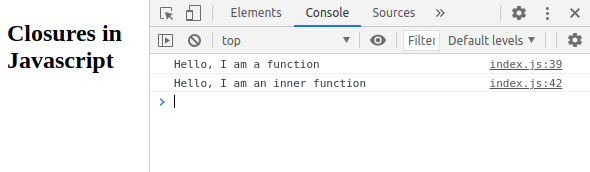Example 4:  Here comes the closure part. Let’s say you don’t only have a simple console.log but some variables are also declared inside the function b(). Now, just stop here & think about the output of the code.

## script.js

 `function` `outer() {``  ``var` `x = 5;`` ` `  ``function` `inner() {``    ``console.log(``"Hello, I am an inner function"``);``    ``console.log(``      ``"I am accessing var x of my parent function"``      ``+ ``" when that parent functions execution "``      ``+ ``"context destroyed"``    ``);``    ``console.log(x);``  ``}``  ``return` `inner;``}``const result = outer();``result();`

Explanation:

1. Global Execution context created, variable result and function a() gets space inside the memory.
2. During the thread execution phase, first of all, a() function gets called.
3. A new execution context gets created var x and function b gets space in memory, and just after it returns the function b which means we have the result as the code of the function, and now the function a() gets removed from the call stack also it’s execution context destroyed.
4. In the last line, when we call function result(), it successfully starts executing the function code inside it. But as soon as it encounters a variable that is not inside the execution context of that function, so it tries to find out the var x in the outer scope but wait, we don’t have access to the execution context of function a (), so how does this x will be executed?
5. Does it mean that var x will become undefined? No, because this is the concept of closure whenever we expose or return the function from another function, not only the code being returned but it comes along with a special thing called the lexical environment, which means the surrounding environment of its outer parent function.
6. So the function b() when returned from the function, it comes along with the lexical environment of its parent hence always has access to the reference of variable x. Note the last line “always have access to the reference of variables” not the value.

Output: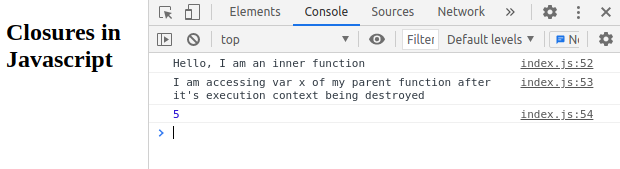Definition: A closure is the combination of functions bunched together with its lexical environment. The lexical environment is the local function memory & the reference to the lexical environment of a parent. In other words, closure is created when a child function keep the environment of the parent scope even after the parent function has already been executed.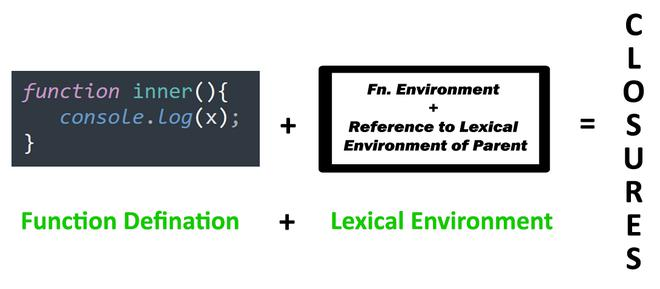This reference to the lexical environment of a parent is the reason why closure functions have access to the variables of outer functions even if those functions are not in the call stack or we can say even if the outer functions are closed.

How closures are created: Closure gets created whenever some function is being created at the time of the creation of another function. In the above example, the function inner is being created at the time of the creation of function a().

Few Usecases & Examples of Closures:

1. Privacy: Whenever we have to hide some functionality or variable as encapsulation, we wrapped that thing inside the lexical environment of the parent.

Example: The below example is a basic template of encapsulation with closures.

Explanation:

1. All the global execution context will be created in the memory allocation phase, the function outer() & the const closure function will get space in memory.
2. At the time of thread execution, the code inside function outer will starts executing because it is the first line in code.
3. Now another execution context will be created and the const notAccessibleByAnyone will get space and later the outer functions return the inner function code and the execution context will vanish out. Notice that the const variable is not in the execution context.
4. But closure function has the reference to that variable, so this is the whole concept of encapsulation with closures.

## script.js

 `function` `outer(secret) {``  ``const notAccessibleByAnyone = secret;`` ` `  ``return` `function` `inner() {``    ``console.log(notAccessibleByAnyone);``  ``};``}`` ` `const closureFunction = outer(``"Secret Data"``);``closureFunction();``console.log(notAccessibleByAnyone);`

Output: In the first line, Secret Data is being printed when accessed by the closure and in the next line ReferenceError is being thrown by javascript when anyone from outside tries to access that.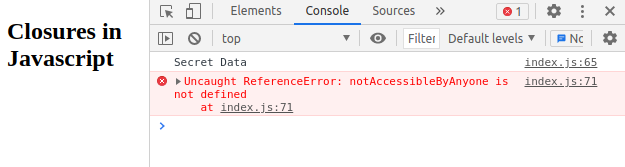2. Partial Functions: When we have to create some kind of functionality in which there exists a common pattern then we create a parent function that takes few parameters and create an inner function that takes fewer parameters than the parent. This concept is also called higher-order functions in which we return functions from function.

Example: The below example provides a basic illustration of how this partial function looks like.

Explanation:

1. Here we have created a parent function that receives a multiplier as a parameter and then passes it to an inner function.
2. Later when we invoke the parent function it returns the code of the inner function which always have the access to that multiplier provided during the parent function call.
3. The function multiplyBy2() holds a function that takes an array and runs for loop on it later returns the modified array in which elements are being multiplied by 2.
4. The function multiplyBy4() holds a function that takes an array and runs for loop on it later returns the modified array in which elements are being multiplied by 4.

## script.js

 `function` `partialApplication(multiplier) {``  ``return` `function` `inner(array) {``    ``let size = array.length;``    ``for` `(let i = 0; i < size; i++) {``      ``array[i] = array[i] * multiplier;``    ``}``    ``return` `array;``  ``};``}`` ` `const multiplyBy2 = partialApplication(2);``const arr1 = multiplyBy2([1, 2, 3]);``console.log(arr1);`` ` `const multiplyBy4 = partialApplication(4);``const arr2 = multiplyBy4([1, 2, 3]);``console.log(arr2);`

Output: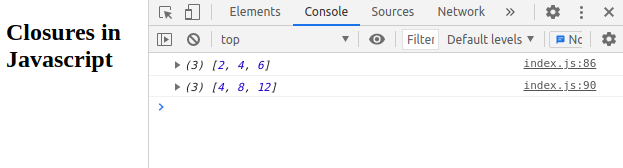My Personal Notes arrow_drop_up